MEDIUM
DATA STRUCTURES AND ALGORITHMS

# How to Solve Insert Delete getRandom O(1)

Written ByTom WagnerYibo Gong

## Insert Delete getRandom O(1) Introduction

The Insert Delete getRandom O(1) problem involves implementing a class that enables the insertion, deletion, and retrieval of a random element in constant time. Solving this design task requires a strong understanding of the operational time complexities for arrays and hash maps.

## Insert Delete getRandom O(1) Problem

Design and implement an efficient sampler that can handle the following operations in constant time:

• `RandomizedSet()` initializes the RandomizedSet object.
• `insert(string val)` Inserts an item `val` into the set if not already present; returns `true` if the item was not present, `false` otherwise.
• `remove(string val)` Removes an item `val` from the set if present; returns `true` if the item was present, false otherwise.
• `getRandom()` Returns a random element from the current set of elements.

Implement the functions of the class such that each function works in average `O(1)` time complexity.

## Example Inputs and Outputs

### Example 1

Input: ["RandomizedSet", "insert", "remove", "insert", "getRandom", "remove", "insert", "getRandom"]

[[], ['a'], ['b'], ['b'], [], ['a'], ['b'], []]

Output: [null, true, false, true, 'b', true, false, 'b']

Explanation:

• `RandomizedSet`: initialize the object
• `insert('a')`: inserts `a` into the set. Returns `true` as it is inserted successfully.
• `remove('b')`: removes `b` from the set. Returns `false` as it does not exist in the set.
• `insert('b')`: inserts `b` into the set. Returns `true` as it is inserted successfully.
• `getRandom()`: returns either `a` or `b` randomly.
• `remove('a')`: removes `a` from the set. Returns `true` as it is removed successfully.
• `insert('b')`: inserts `b` into the set. Returns `false` as it already exists in the set.
• `getRandom()`: returns `b` as it is the only item in the set.

Constraints

• At most 2 * 10^5 calls will be made to `insert`, `remove`, and `getRandom`.
• There will be at least one element in the data structure when getRandom is called.

## Insert Delete getRandom O(1) Solutions

When writing code to store data we have lots of data structures to choose from: arrays / lists, trees, graphs, hashmaps, linked lists, sets, etc. And different data structures have different time complexities for various operations, so depending on what we are trying to achieve, the “right” data structure to use will change. In this problem, we need to achieve `O(1)` for `insert`, `remove` and `getRandom`. Because of this requirement, let’s focus on two widely used data structures that have certain `O(1)`operations: hashmaps and arrays.

• Hashmap
• add a key: `O(1)`
• read a given key: `O(1)`
• delete a given key: `O(1)`
• read a random key: `O(N)`
• get all the keys in the hashmap: `O(N)`
• get a random key from the keys: `O(1)`
• Array
• add an item to the end of the array: `O(1)`
• read the value without providing an index: `O(N)`
• delete an item from the end of the array: `O(1)`
• delete an item from the middle of the array: `O(N)`
• need to create a new array and copy all the items from the old array (except the item to be deleted) into the new array: `O(N)`
• get a random item: `O(1)`
• get a random index within the range of the array length: `O(1)`
• read the value for the random index: `O(1)`

Back to our problem at hand, based on the time complexities above we can use a hashmap to achieve `O(1)` inserts and deletes, but a hashmap alone will not be sufficient for getting a random element. Likewise, we can use an array to get a random element but cannot use an array alone for `O(1)` reads and deletes. Since there is no limitation on space usage, we can combine both data structures in our implementation:

1. Use a hashmap to memorize the positions for all the items in the array to achieve `O(1)` read and delete.

2. Use an array to get a random element with `O(1)`.

#### Approach 1: Hashmap + Array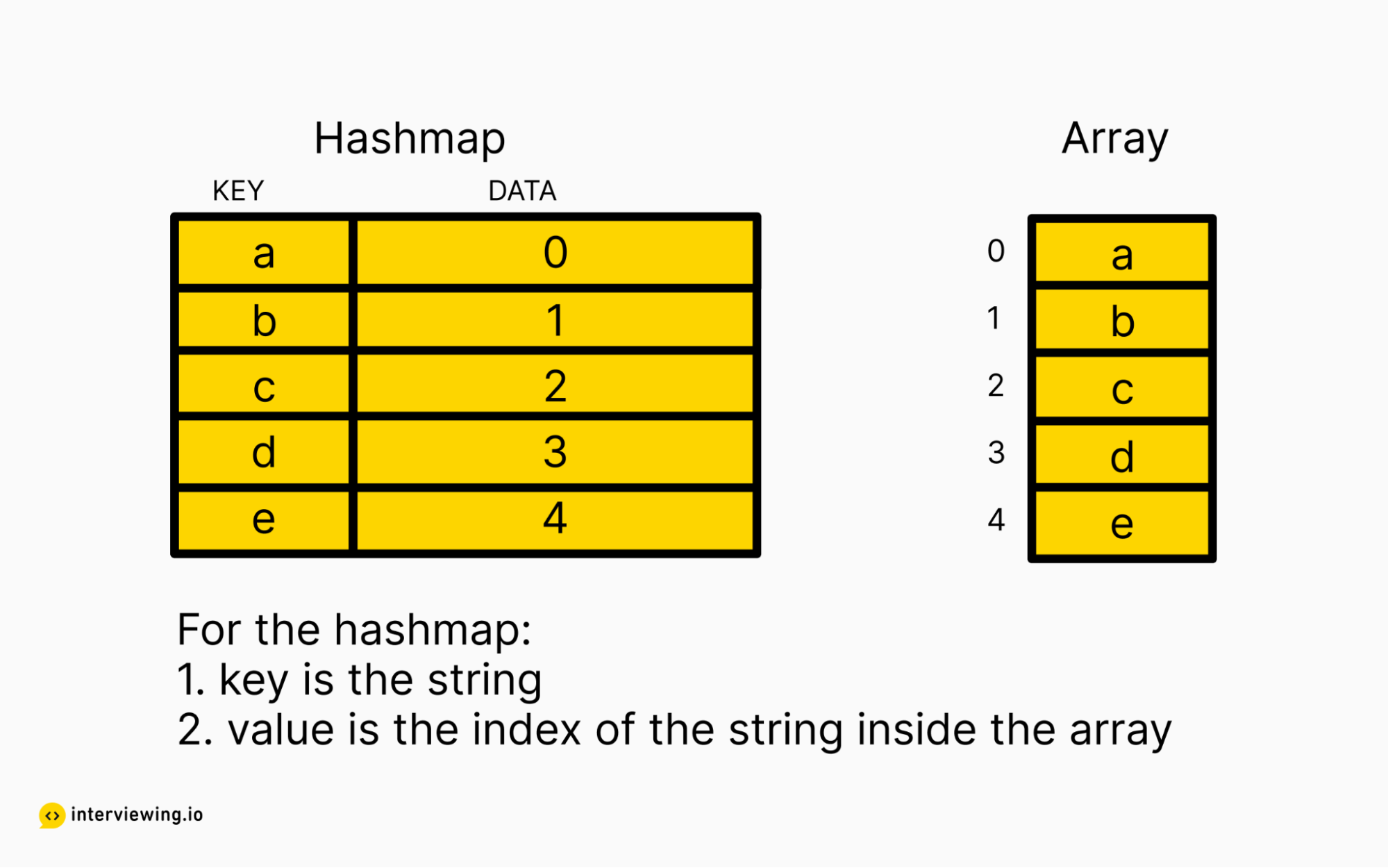As shown in the diagram above, we will use two objects to store the strings:

• An array to store all the strings we currently have.
• A hashmap, with the key as the string and value as the index of the string in the array, so that we can keep track of the positions of the strings inside the array.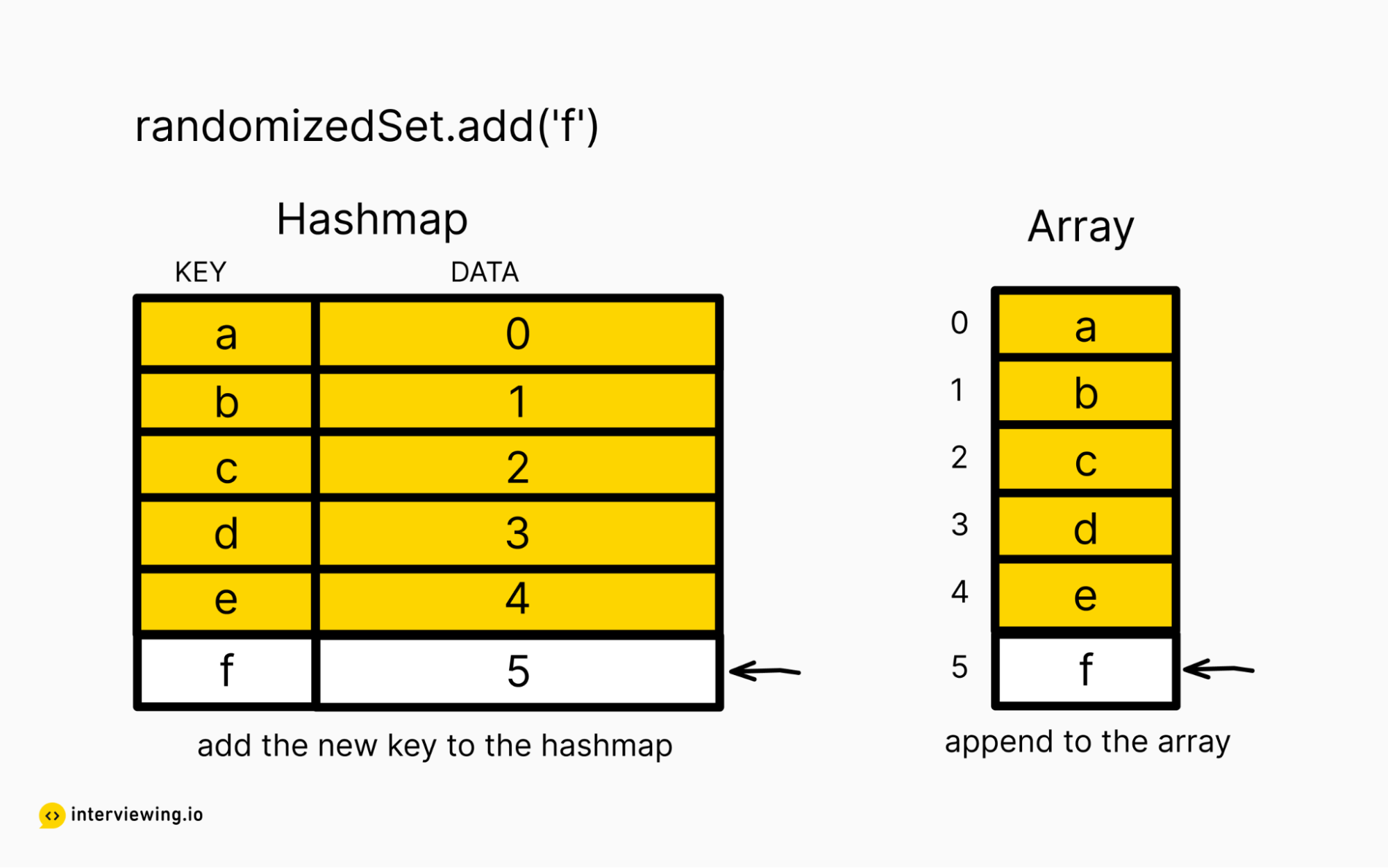For `add` operations, we can:

• Check if the string exists in the hash map or not; return `false` if we already have the string.
• Append the string to the end of the array. The index of the newly-added string will be `array_length - 1`. In our example, we append `f` to the end of the array and the new array length is `6`, which means `f` is stored at index `5`.
• Add the string into the hashmap with the key as the string itself, and the value as the index. In our example, we add `f` into the hashmap with a value of `5`.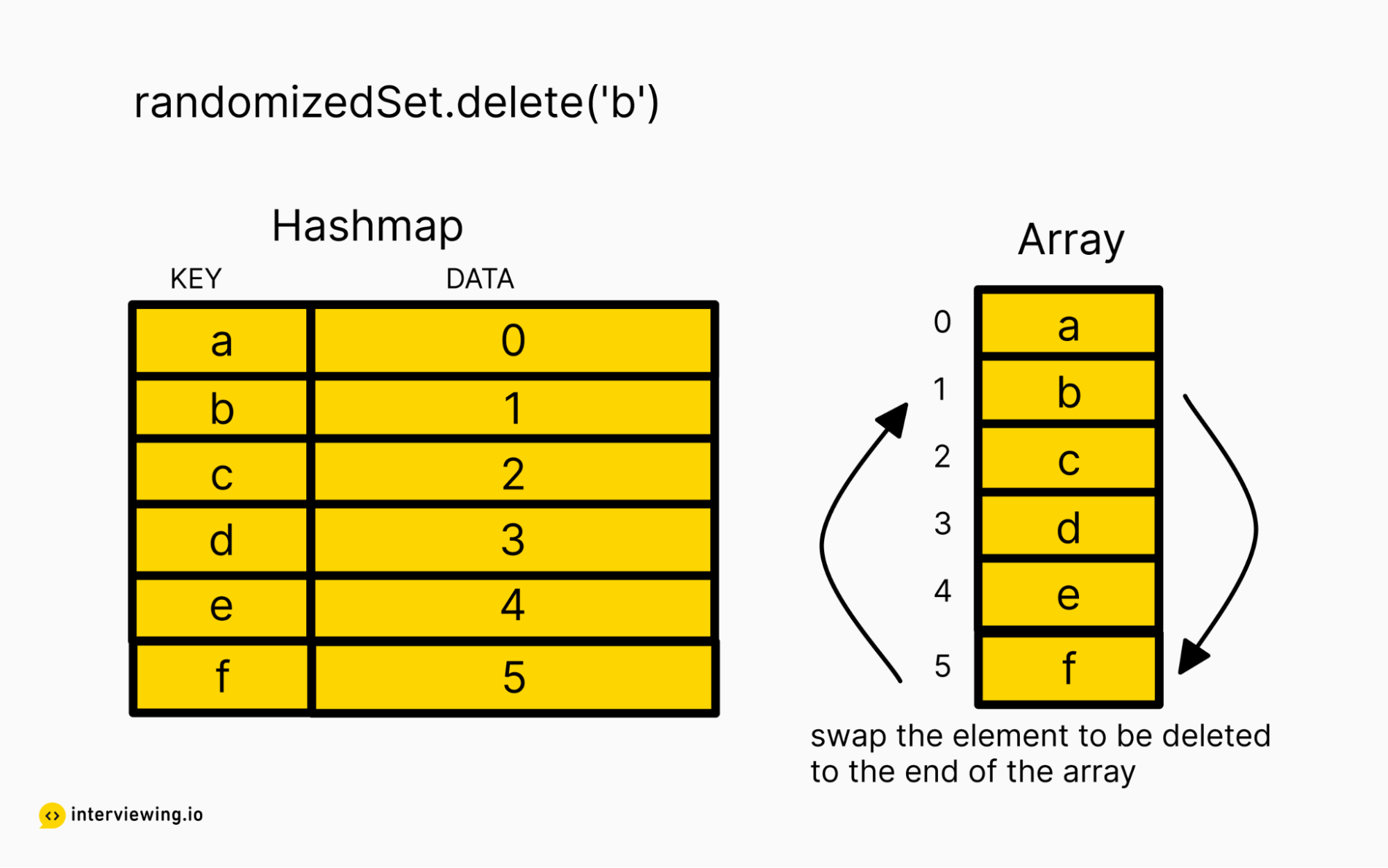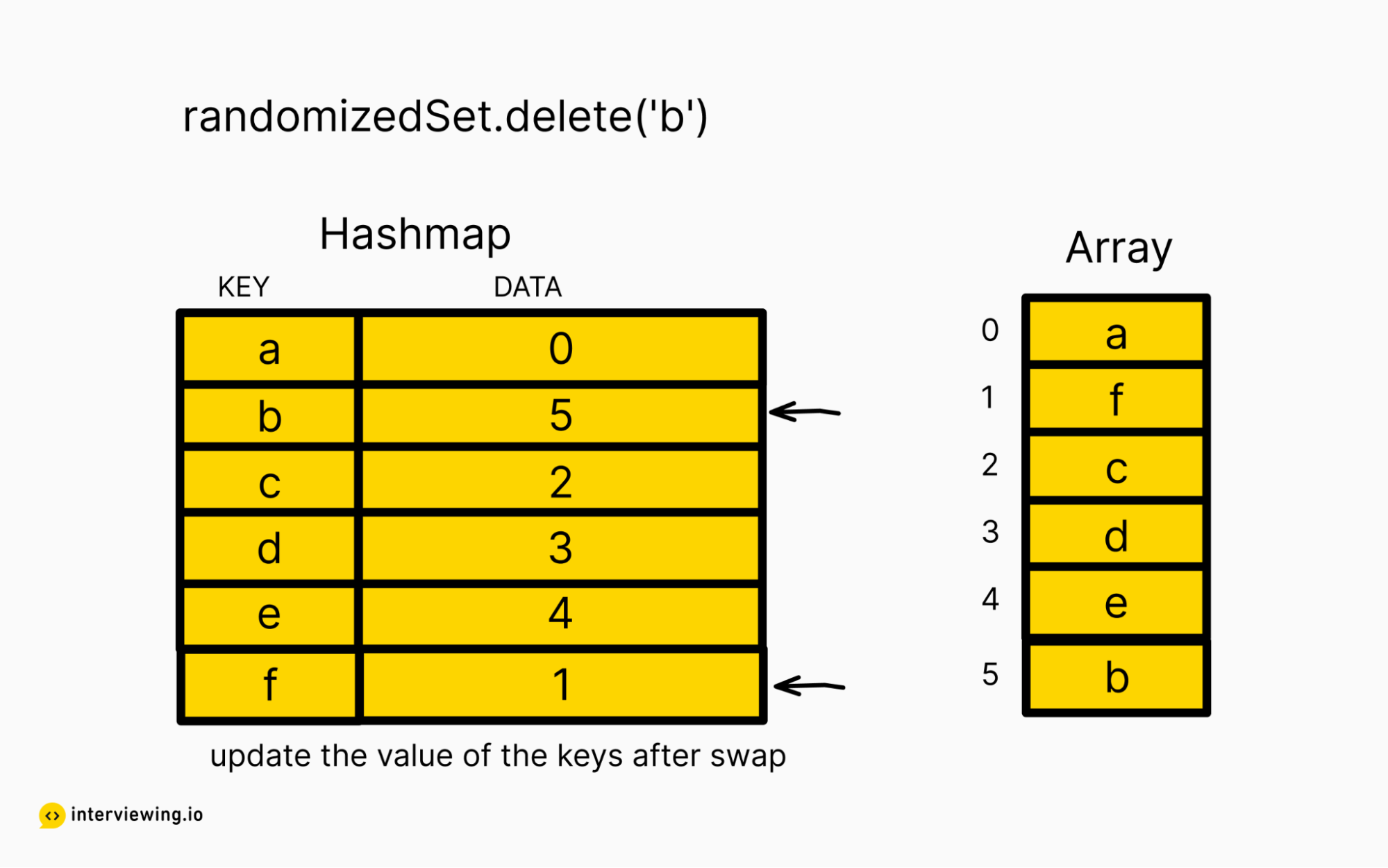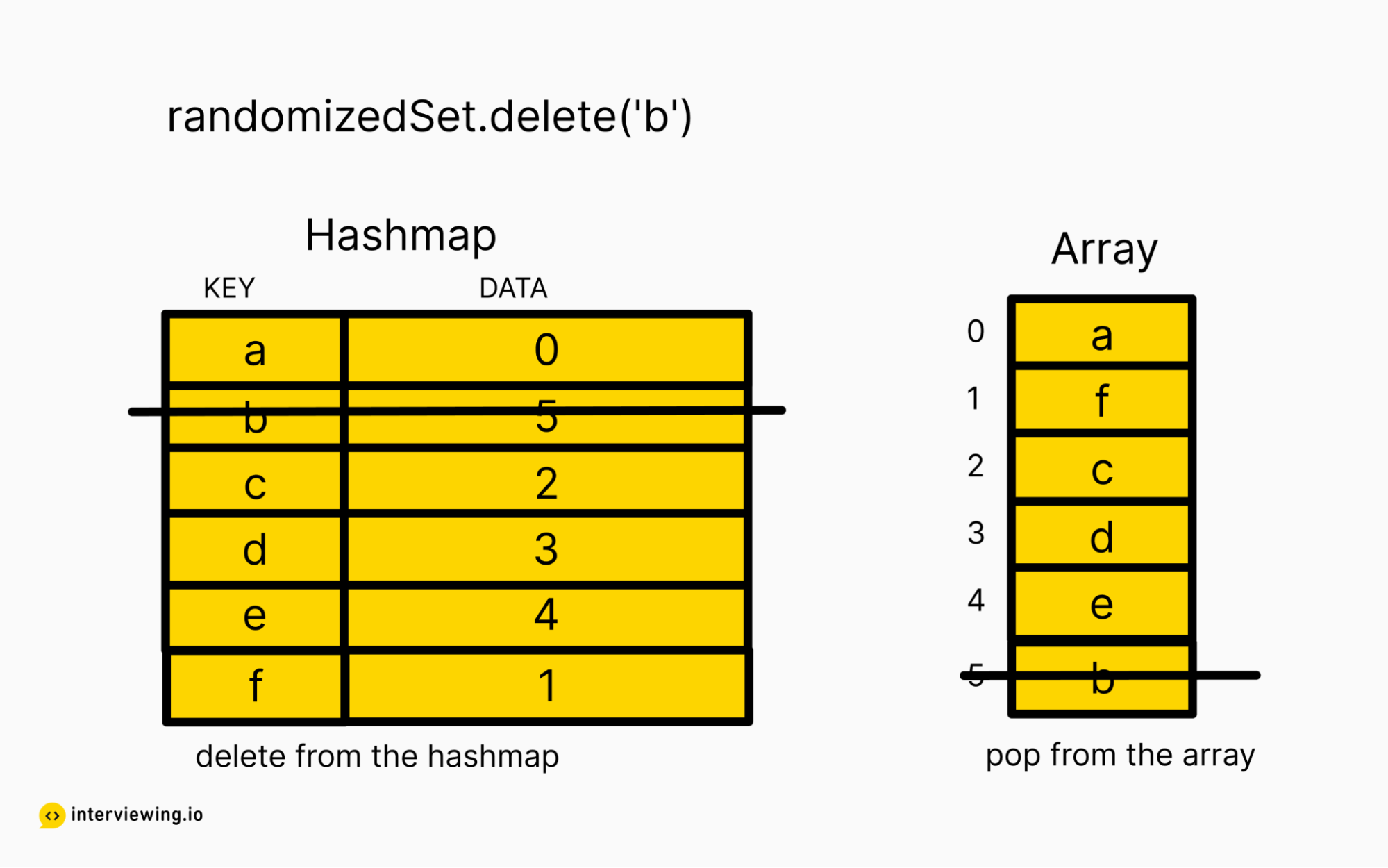For `delete` operations, we can:

• Get the index of the string to be deleted from the hashmap. In our example, we are deleting `b` and we retrieve the array-index of `b` from the hashmap, which is `1`.
• We can then swap the string to be deleted with the last element in the array. In our example, we move `b` to the end of the array, and move the last element (`f`) to index `1` in the array.
• Next, we need to update the keys in the hashmap with their new array index values. In our example, we need to update `b` to point at `5` and `f` to point at `1`.
• Lastly, we pop the last element from the array and delete the key from the hashmap.

For `getRandom` operations, we can:

• Get the length of the array, knowing the last index of the array is `array_length - 1`.
• Generate a random number (`randomIdx`) in the range between `0` (the first index) and the last index (inclusive).
• Return the element stored at the random index in the array.

#### Insert Delete getRandom O(1) Python Solution - Hashmap + Array

``````from random import randint

class RandomizedSet(object):

def __init__(self):
# Key is the string, value is the index of the string inside the array
# Use this hashmap to keep track of the position of the string in the array
self.item_to_index = {}

# The array of strings we have inserted
self.item_arr = []

def insert(self, item):
# Only insert if the string does not already exist
if item in self.item_to_index:
return False

# Append this new string to the end of the array
index = len(self.item_arr)
self.item_to_index[item] = index
self.item_arr.append(item)

return True

def remove(self, item):
# Only remove if the string exists
if item not in self.item_to_index:
return False

# Swap the string to be removed to the end of the array
index = self.item_to_index[item]
last_item = self.item_arr[-1]
self.item_to_index[last_item] = index
self.item_arr[index] = last_item
self.item_arr.pop()
del self.item_to_index[item]

return True

def getRandom(self):
# Randomly pick an index available
index = randint(0, len(self.item_arr) - 1)
return self.item_arr[index]
``````

#### Time/Space Complexity

• Time complexity: `O(1)` for all the operations.

• Space complexity: `O(N)`

• The hashmap takes `O(N)`
• The array list takes `O(N)`

## Practice the Insert Delete getRandom O(1) Problem With Our AI Interviewer

Start AI Interview